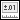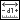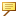To Set the Dimension Bounds
When you design a model, you expect the actual part dimensions to be within certain predetermined limits of size. Creo Parametric defines these limits of size—upper and lower dimension boundaries—as dimension bounds. The system creates model geometry based on nominal values for each dimension.
You can create a hypothetical model of a part or assembly based on upper and lower allowable dimensions, and later use this new geometry to measure the effects of various analysis functions.
1. Click Analysis and then click the arrow next toTolerance Analysis.
2. ClickDimension Boundaries. The DIM BOUNDS menu appears.
3. On the DIM BOUNDS menu, do one of the following:
Choose Set All to set all part dimensions to the specified boundary (in Assembly mode, select parts or subassemblies).
Choose Set Selected to set only selected dimensions to the specified boundary.
4. Specify the value limits by choosing one of the following commands:
Upper—Sets the dimension value to its maximum (generates geometry based on a nominal dimension value plus the tolerance).
Middle—Sets the dimension value to the nominal value plus the mean of the upper and lower tolerance values.
Lower—Sets the dimension value to its minimum (generates geometry based on a nominal dimension value minus the tolerance).
Nominal—Sets the dimension value to nominal (generates geometry based on exact ideal dimensions).
5. Select part features to display dimensions whose boundaries must be set.
6. Select the dimensions.
7. Click Done to accept the dimension bounds, or click Quit to abort.
8. To reset the part to its original state, click Set All > Nominal on the DIM BOUNDS menu.When you modify dimension bounds, the system generates geometry based on new dimensions. To reflect these changes, it updates reference dimensions present in the model accordingly. The part dimensions appear grey until the dimension bounds are set back to normal.